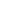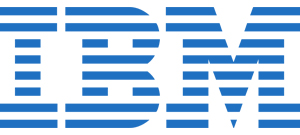18756 Stone Oak Park Way, Suite200, San Antonio TX 78258 USA
100 Queen St W, Brampton, ON L6X 1A4, CanadaUnited States# IBM SPSS Statistics Essentials (v25) Training

###### What IBM SPSS Statistics Essentials (v25) training is all about?

IBM SPSS Statistics Essentials (v25) Training is aimed at professionals to learn more about the fundamentals of using IBM SPSS Statistics for the classic data analytics process. This technical course educates individuals on the fundamentals of the data definition, data modification, reading data, data assessment and presentation of analytical results. Our enterprise training program is ideal for organizations and companies. It teaches you to transform variables and utilize functions to compute them.

You will learn to merge data files, and it also helps you choose cases for analyses and summarize individual variables. This program is suitable for professionals who want to get familiar with IBM SPSS Statistics program and individuals who are thinking of purchasing it. Through this course, you will learn various essential aspects, including customizing pivot tables, working with syntax, and chart customization and creation.

###### Schedule

You can call us on 1-800-961-0337 or Chat with our representative.

###### What are the course objectives for IBM SPSS Statistics Essentials (v25) training?
• Brief introduction of IBM SPSS Statistics.
• Transforming the variables.
• Utilizing functionalities to compute the variables.
• Setting the unit analysis.
• Merging the data files.
• Choosing cases for analysts.
• Summarizing the individualsâ€™ variables.
• Describing the relationships between the variables.
• Creating the presentation ready tables covering customized tables.
• Customizing the pivot tables.
• Customizing and creating charts.
• Functioning with the syntax.
• Controlling the IBM statistics environment.
###### Who should attend IBM SPSS Statistics Essentials (v25) training?

This learning is designed for data analysts having minimum experience in IBM SPSS. Moreover, the target audience for this training are Business analysts and BFFs can also sign for this course.

###### What is the course outline for IBM SPSS Statistics Essentials (v25) training?
• 1. Introduction to IBM SPSS Statistics
• a). Review basic concepts in IBM SPSS Statistics
• b). Identify the steps in the research process
• c). Review basic analyses
• d). Use Help
• a). Overview of data sources
• b). Read data from databases
• c). Read data from Microsoft Excel
• d). Define variable properties
• 3. Transform variables
• a). Compute new variables
• b). Recode values of nominal, ordinal, and scale variables
• c). Create a numeric variable from a string variable
• d). Explore advanced variable transformations
• 4. Use functions to compute variables
• a). Use statistical functions
• b). Use logical functions
• c). Use missing value functions
• d). Use conversion functions
• e). Use system variables
• f). Use the Data and Time Wizard
• 5. Set the unit of analysis
• a). Remove duplicate cases
• b). Create aggregated datasets
• c). Restructure datasets
• 6. Merge data files
• a). Add cases from one dataset to another
• b). Add variables from one dataset to another
• c). Enrich a dataset with aggregated information
• 7. Select cases for analyses
• a). Select case for an analysis
• b). Run analyses for subgroups
• 8. Summarize individual variables
• a). Define levels of measurement
• b). Summarizing categorical variables with Frequencies
• c). Summarizing scale variables with Frequencies and Descriptives
• 9. Describe relationship between variables
• a). Select the appropriate procedure to summarize the relationship between two variables
• b). Summarize the relationship between categorical variables with Crosstabs
• c). Summarize the relationship between a scale and a categorical variable with Means
• 10. Create presentation-ready tables with Custom Tables
• a). Identify table layouts
• b). Create tables for variables with shared categorical
• c). Create tables for variables that represent a multiple response question
• 11. Customize pivot tables
• a). Perform Automated Output Modification
• b). Customize pivot tables in the Pivot Table Editor
• c). Use table templates
• d). Export pivot tables to other applications
• 12. Create and customize charts
• a). Use the Chart Builder to create various types of graphs
• b). Customize charts in the Chart Editor
• c). Use chart templates
• d). Export pivot tables to other applications
• 13. Work with syntax
• a). Use syntax to automate analyses
• b). Use the Syntax Editor environment
• c). Create and edit syntax
• d). Run syntax
• 14. Control the IBM SPSS Statistics environment
• a). Describe the features of the Options dialog box
• b). Reset the display of variables
• c). Set the default working data folders2 Days | \$ 1590